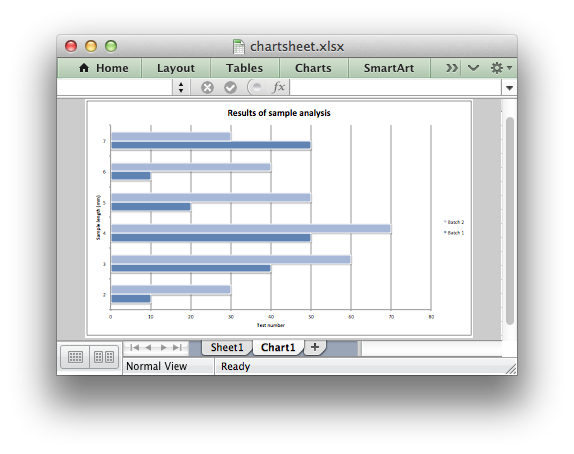# Example: Chartsheet

Example of creating an Excel Bar chart on a chartsheet.```#######################################################################
#
# An example of creating an Excel chart in a chartsheet with Python
# and XlsxWriter.
#
# Copyright 2013-2023, John McNamara, jmcnamara@cpan.org
#
import xlsxwriter

workbook = xlsxwriter.Workbook("chartsheet.xlsx")

# Add a worksheet to hold the data.

# Add a chartsheet. A worksheet that only holds a chart.

# Add the worksheet data that the charts will refer to.
headings = ["Number", "Batch 1", "Batch 2"]
data = [
[2, 3, 4, 5, 6, 7],
[10, 40, 50, 20, 10, 50],
[30, 60, 70, 50, 40, 30],
]

worksheet.write_column("A2", data)
worksheet.write_column("B2", data)
worksheet.write_column("C2", data)

# Create a new bar chart.

# Configure the first series.
{
"name": "=Sheet1!\$B\$1",
"categories": "=Sheet1!\$A\$2:\$A\$7",
"values": "=Sheet1!\$B\$2:\$B\$7",
}
)

# Configure a second series. Note use of alternative syntax to define ranges.
{
"name": ["Sheet1", 0, 2],
"categories": ["Sheet1", 1, 0, 6, 0],
"values": ["Sheet1", 1, 2, 6, 2],
}
)

# Add a chart title and some axis labels.
chart1.set_title({"name": "Results of sample analysis"})
chart1.set_x_axis({"name": "Test number"})
chart1.set_y_axis({"name": "Sample length (mm)"})

# Set an Excel chart style.
chart1.set_style(11)

# Add the chart to the chartsheet.
chartsheet.set_chart(chart1)

# Display the chartsheet as the active sheet when the workbook is opened.
chartsheet.activate()

workbook.close()
```